# Team:Aberdeen Scotland/Stochastic Model

University of Aberdeen - ayeSwitch - iGEM 2010

iGEM 2010

# The Stochastic Model

Stochastic modelling can be used when modelling biochemical processes and intracellular dynamics . It is also widely used in many other areas such as physics, economics, geophysical systems and even music.

### Overview of the Deterministic Model

A first look at how our system behaved involved modelling it deterministically. The deterministic model is based on constructing a set of reaction-rate equations to describe the biochemical pathways being studied. These equations are non-linear ordinary differential equations (ODEs). The concentrations of chemical species are the variables and the parameters are the reaction rate constants. Solving the ODEs gives how the resulting concentrations of the chemical species change in time ,.

In a deterministic system, the time evolution is considered to be continuous and predictable. Strictly speaking, the time evolution of a chemically reacting system is not a continuous process because molecular population levels can only change by discrete integer amounts. In order to predict the molecular population levels at a future time by means of a deterministic approach, we have to take into account the precise positions and velocities of all the molecules in the system , which becomes practically impossible.

In general, concentrations are only defined for large numbers of molecules. In this situation, when numbers change by one or two units in a reaction, these changes can be treated differentially. Also, when the number of molecules is large, any two reactions can take place at the same time. The system of ODEs thus represents a collection of reactions occurring simultaneously .

The deterministic model has some disadvantages however. It is unable to describe the fluctuations in the molecular population levels, which can become very important if the numbers of the molecules involved in the reactions are very low .

### Introducing the Stochastic Model

The stochastic model is a way of following the dynamics of individual molecules . In addition to the deterministic simulation, we also perform the stochastic simulation to see if the potential fluctuations due to the low numbers of molecules involved in our system play a major role. We assume that reactions cannot happen simultaneously and that they do not happen continuously throughout time. There is also now a probability attached to when a reaction will happen and which reaction will occur.

We consider the time evolution of a chemical system to be a discrete process instead of a continuous, deterministic process. The stochastic model thinks of the time evolution as a random process with probabilities as variables ,. The stochastic model involves the same decomposition of a pathway into elementary reactions. However, here we are looking at numbers of molecules instead of concentrations . The connection with molecular concentrations appears when, in the stochastic model, averages are taken over many cells. These averages satisfy the same equations as the concentrations. Thus the behaviour of concentrations can be interpreted as that of a population average, provided that fluctuations around the average are small .

### How to Model Systems Stochastically

Modelling a system of differential equations stochastically can be done using the Gillespie Algorithm which was devised by Daniel T. Gillespie in 1977 . However, Gillespie also devised a variation on his method which is known as the tau-leaping method. This method results in faster simulations because it is less exact than the actual Gillespie Algorithm as it uses much larger time steps and therefore has less calculations to perform.

Due to time constraints we modelled our system using the tau-leaping model.

The tau-leaping method proceeds as follows:

1. Specify a time step, tau.
2. Multiply each term of each differential equation by tau.
3. Apply Poisson distribution function to each result from step 2.
4. Generate a random number using the Poisson distribution created in step 3.
5. Calculate the new number of molecules by adding or subtracting each random number from step 4, from the initial number of molecules. Adding if the change has a positive influence on the system, subtracting if it has a negative influence on the system.
6. Repeat steps 1-5 for a specified number of reactions, N.

This algorithm allowed us to view what our model should look like stochastically. The only problem is that we do not know exactly how many molecules we are starting with. However, this is a problem which affects the deterministic model as well as the stochastic model. In both models, the initial concentrations or number of molecules needs to be specified. Until we know how many molecules we begin with, this program will only give us an idea of how our system could evolve. We decided to start our simulations with only one molecule of each of the mRNAs and proteins and watch how the system evolved from there.

### Results

The deterministic model describes the average change in concentration over time. Since the stochastic model deals with individual particles we would expect the stochastic model to follow the trend of the deterministic model but there would be fluctuations around the average value. If we were to take the average of these particles over time, it would look like the deterministic model. The results showed exactly what we expected. The stochastic model follows the trend set by the deterministic model but it fluctuates, sometimes wildly, around the average. One thing to note is that the deterministic model sometimes suggests that we will not see bistability. However, because of the fluctuations around the average in the stochastic model, one fluctuation could push us into the realm of bistability. In the pictures below the stochastic model is on the left and the deterministic model is on the right. One can clearly see the similarity in the overall shape and it is clear that the deterministic model is just the average of the stochastic model.

Scenario 1: GAL and METH present, winner GFP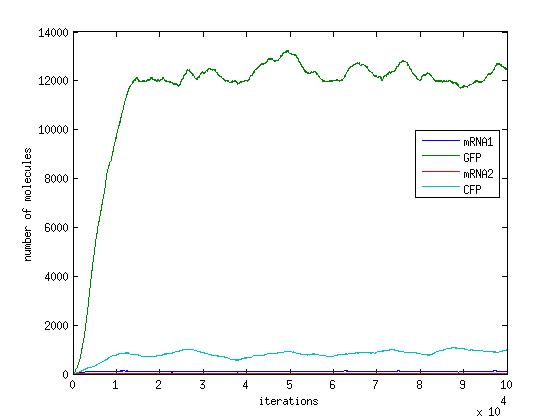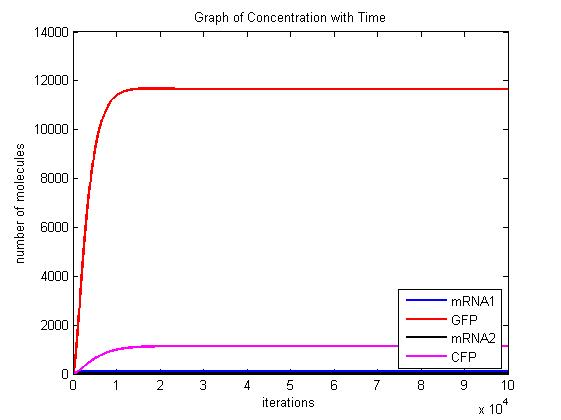When both galactose and methionine are present, the methionine inhibits the production of the CFP and the galactose encourages the production of the GFP. With nothing to inhibit the production of GFP, the GFP dominates.

Scenario 2: no METH and GAL present, winner – either, depends on other parameters!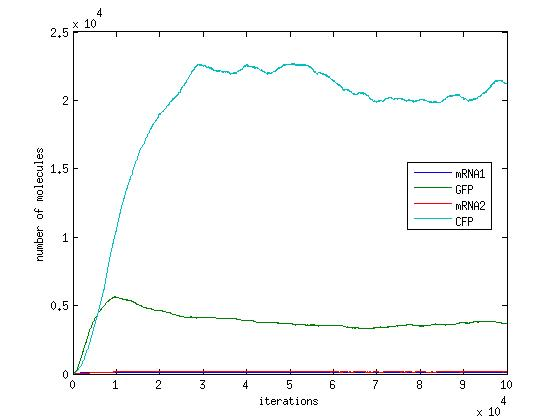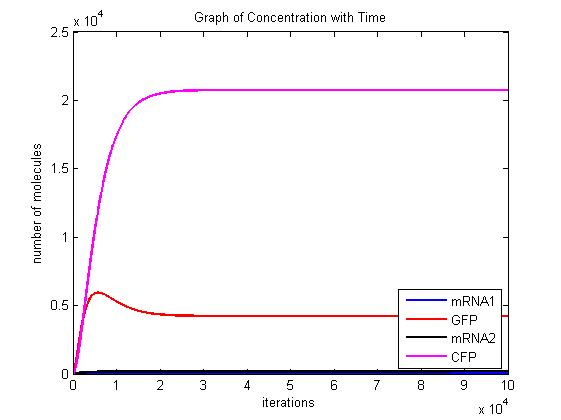When no methionine is present, CFP can be produced. Also, when GAL is present GFP can be produced. When both proteins are produced, they will both inhibit each other. In this scenario, the dominant protein will depend on the parameters of the system. The modelling we have done suggests that the parameters which affect the system are the transcription/translation rates (λ values) and the binding coefficients (K values). As shown in the above graphs, the parameters of our system dictate that CFP will win.

Scenario 3: no METH and no GAL present, winner CFPNo methionine present leads to CFP being produced. No galactose present means that no GFP will be produced. With nothing to inhibit the CFP production, CFP will dominate.

Also, a simulation was run with variable galactose and methionine over time. The aim of this was to model the switching behaviour of our system. The picture below shows the results of the stochastic simulation. What we see, is a clear switching behaviour, but only if we actively remove one protein before adding the other.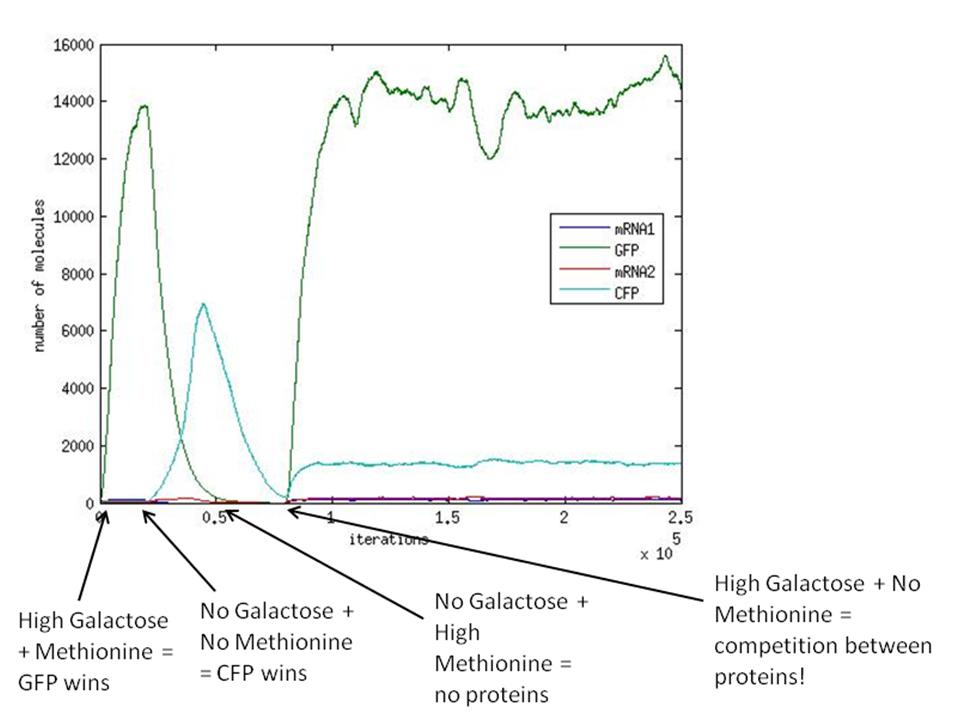As mentioned previously, when GAL and no METH are present in the system (at 80000 iterations), the winning protein will depend heavily on the parameters of the system. Our experience has shown us that the values for the transcription/translation rates (λ values) and the dissociation constants (K values) strongly affect how the system behaves and thus determines what protein will dominate.

### Conclusions

The stochastic model is a more accurate method of modelling a biochemical system where low numbers of molecules are involved. The stochastic model follows the actions of individual molecules whereas the ODEs based model describes how concentrations change continuously in time. The deterministic model is basically just the average of the stochastic model and does not represent any fluctuations due to the individual molecules present.

### References

 Gillespie, D.T. (1977), Exact Stochastic Simulation of Coupled Chemical Reactions, The Journal of Physical Chemistry, Vol. 81, No. 25.

 Ullah, M., Schmidt, H., Cho, K.-H. and Wolkenhauer, O. (2006), Deterministic modelling and stochastic simulation of biochemical pathways using MATLAB, IEE Proc.-Syst. Biol., Vol. 153, No. 2.

 Hayot, F. (2008), Single Cell experiments and Gillespie’s algorithm. Retrieved from http://tsb.mssm.edu/summerschool/images/4/4d/HayotSlides.pdf

 Department of Computational & Applied Mathematics, Rice University, Modeling and Simulation of Reaction Networks. Retrieved from http://www.caam.rice.edu/~caam210/reac/lec.html

Back to the Top